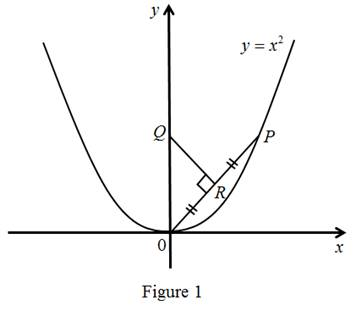# The position of Q when P approaches to origin and the limiting position of Q .### Single Variable Calculus: Concepts...

4th Edition
James Stewart
Publisher: Cengage Learning
ISBN: 9781337687805### Single Variable Calculus: Concepts...

4th Edition
James Stewart
Publisher: Cengage Learning
ISBN: 9781337687805

#### Solutions

Chapter 2, Problem 4P
To determine

## To find: The position of Q when P approaches to origin and the limiting position of Q.

Expert Solution

The limiting position of Q is (0,12)_.

### Explanation of Solution

Result used:

Slope of Perpendicular lines: The product of slope of the two perpendicular lines equal to –1.

Graph:Calculation:

The perpendicular bisector of OP intersects the y-axis as P approaches the origin along the parabola then the length of the Q will increases and joins at a point R on the parabola.

Let R be the midpoint of OP, since P(x,x2) then R(x2,x22).

Let Q(0,a) and from figure 1that is OPQR.

The slope of OP is

mOP=x20x0=x2x=x

By the slopes of the perpendicular lines

mQR×mOP=1mQR=1mOP

mQR=1x (1)

Let Q(0,a),R(x2,x22) bet the two points then the slope of QR is

mQR=x22ax20=x22a2x2

mQR=x22ax (2)

From equations (1) and (2)

1x=x22ax1=x22a2a=x2+1a=x22+12

In above equation if x approaches 0,

a=(0)22+12a=12

Thus the required limiting position of Q is (0,12)_.

### Have a homework question?

Subscribe to bartleby learn! Ask subject matter experts 30 homework questions each month. Plus, you’ll have access to millions of step-by-step textbook answers!